### Showing videos fromEddie Woo with a total of 3,991 videos

05:50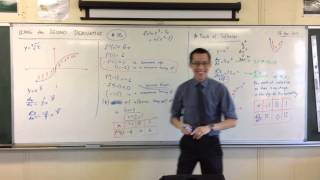Using the Second Derivative (5 of 5: Where the concavity changes but f"(x) doesn't exist)
3y5m ago
05:43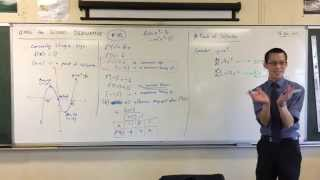Using the Second Derivative (4 of 5: Examples where f"(x)=0 doesn't mean Point of Inflexion)
3y5m ago
07:10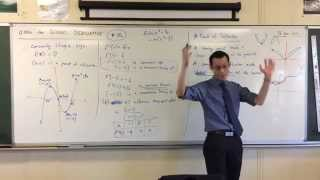Using the Second Derivative (3 of 5: Why the Points of Inflexion may not exist when f"(x) = 0)
3y5m ago
09:12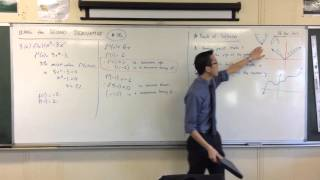Using the Second Derivative (2 of 5: Turning Point vs Stationary Point analogy)
3y5m ago
11:02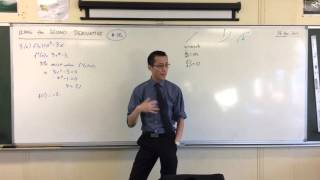Using the Second Derivative (1 of 5: Finding the Point of Inflexion)
3y5m ago
12:14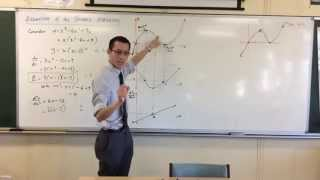Geometry of the Second Derivative (4 of 4: Why does the Derivative Graphs correlate?)
3y5m ago
10:22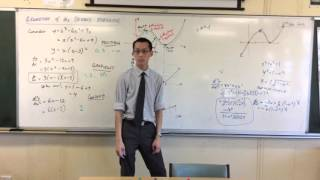Geometry of the Second Derivative (3 of 4: Finding the concavity of a circle)
3y5m ago
08:23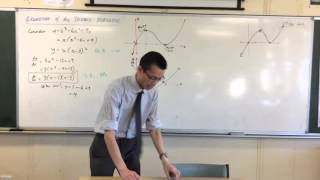Geometry of the Second Derivative (2 of 4: Graphical correspondence of original and its derivatives)
3y5m ago
08:49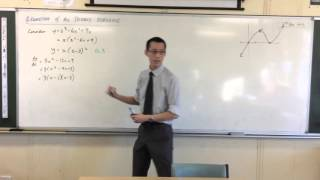Geometry of the Second Derivative (1 of 4: Reviewing the Derivative)
3y5m ago
10:23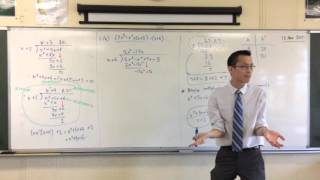Division of Polynomials (2 of 2: Using Long Division to divide Polynomials)
3y5m ago
09:20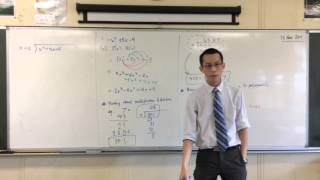Division of Polynomials (1 of : Introduction to Multiplication and Division of Polynomials)
3y5m ago
07:23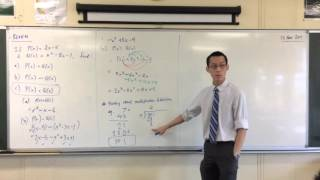Review of Polynomials (2 of 2: Multiplication of Polynomials)
3y5m ago
07:11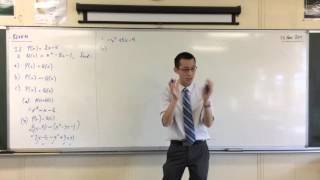Review of Polynomials (1 of 2: The Addition & Subtraction of Polynomials)
3y5m ago
06:40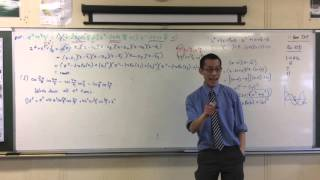Complex Numbers (6 of 6: Finishing off the Proof)
3y5m ago
11:14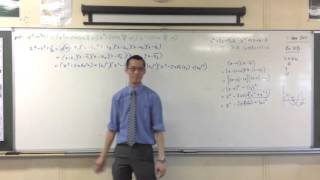Complex Numbers (5 of 6: Complex Numbers Proofs [Using the Conjugate])
3y5m ago
07:12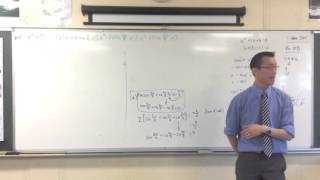Complex Numbers (4 of 6: Harder Complex Numbers Questions) [Student Requested Problem]
3y5m ago
07:54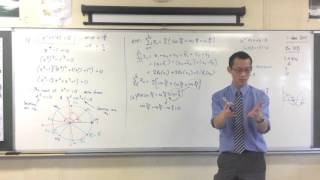Complex Numbers (3 of 6: Harder Complex Numbers Question) [Student Requested Problem]
3y5m ago
06:24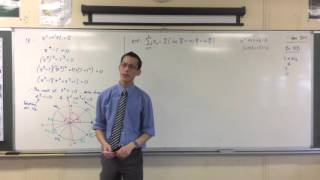Complex Numbers (2 of 6: Solving Harder Complex Numbers Questions) [Student Requested Problem]
3y5m ago
10:36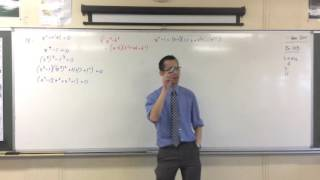Complex Numbers (1 of 6: Solving Harder Complex Numbers Questions) [Student requested problem]
3y5m ago
04:19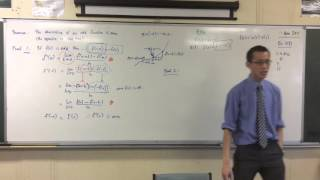The Second Derivative (3 of 3: Using the Product Rule to Prove Theorems)
3y5m ago
07:20The Second Derivative (1 of 3: Introducing Terminology)
3y5m ago
11:41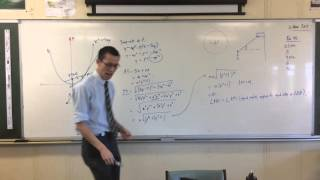HSC Harder Parametrics Question (2 of 2: Breaking down the Question and Solving)
3y5m ago
10:43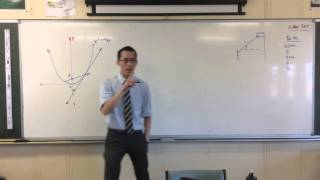HSC Harder Parametrics Question (1 of 2: Introducing the Question)
3y5m ago
06:26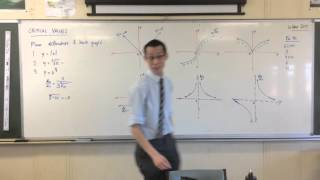Critical Values of Functions (2 of 2: Odd and Even Correspondence in derivatives and primitives)
3y5m ago
12:42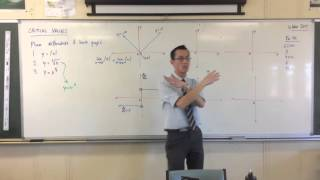Critical Values of Functions (1 of 2: Insights produced by Critical Values)
3y5m ago
07:19Polynomials (2 of 3: Adding & Subtracting Polynomials)
3y5m ago
09:38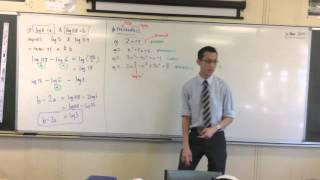Polynomials (1 of 3: Introduction to Polynomials)
3y5m ago
06:16Logarithms (1 of 1: Solving Logs with Pronumerals)
3y5m ago
09:11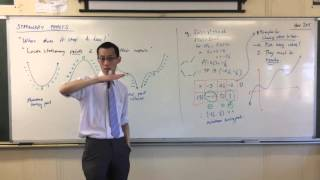Exploring Stationary Points (3 of 3: Principles for Choosing Values to Test for Stationary Points)
3y5m ago
07:34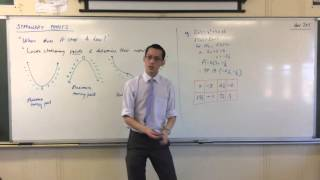Exploring Stationary Points (2 of 3: Introductory Examples regarding nature of Stationary Points)
3y5m ago
08:35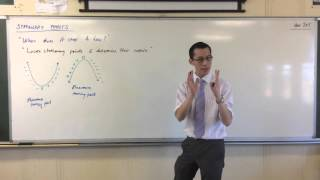Exploring Stationary Points (1 of 3: In Depth Introduction to Stationary Points)
3y5m ago
06:22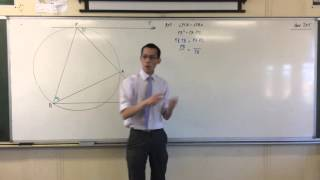HSC Wk6 Quiz Overview (Solving A Harder Circle Geometry Question)
3y5m ago
12:01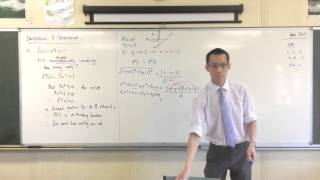Harder Locus Problems (Student Requested Question: Parabola flipped 45*)
3y5m ago
08:03Increasing & Decreasing (1 of 1: Harder Examples)
3y5m ago
10:58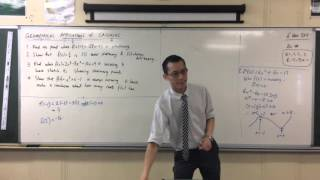Geometrical Applications of Calculus (4 of 4: Using the derivative for stationary points of a graph)
3y5m ago
08:53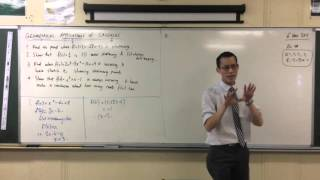Geometrical Applications of Calculus (3 of 4: Some Introductory Examples)
3y5m ago
04:32Geometrical Applications of Calculus (2 of 4: Increasing, Decreasing or Stationary Points)
3y5m ago
09:57Geometrical Applications of Calculus (1 of 4: An Introduction to the applications of calculus)
3y5m ago
12:45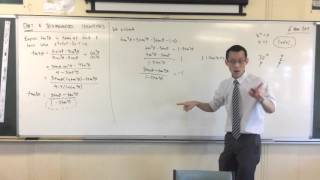DMT and Trig Identities (4 of 4: Using Multi-angle formula to solve polynomials)
3y5m ago
07:52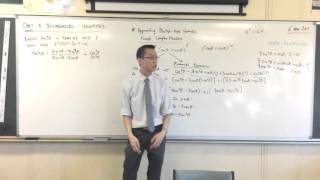DMT and Trig Identities (3 of 4: Deriving tan expression from cos and sin)
3y5m ago
10:54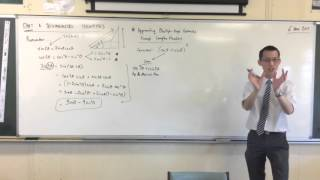DMT and Trig Identities (2 of 4: Using De Moivre's Theorem and Binomial Expansions)
3y5m ago
08:31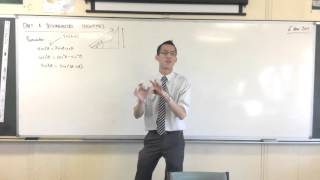DMT and Trig Identities (1 of 4: Deriving multi-angle identities with compound angles)
3y5m ago
06:28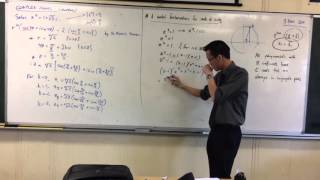Complex Conjugate Root Theorem (4 of 4: Using Factorisation to find patterns with Roots of Unity)
3y5m ago
10:29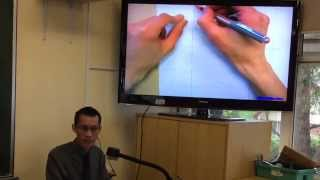Complex Conjugate Root Theorem (3 of 4: Geometrical Shape represented by Conjugate Root Theorem)
3y5m ago
12:22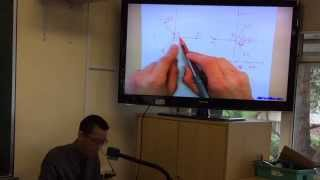Complex Conjugate Root Theorem (2 of 4: Introduction to the Conjugate Root Theorem)
3y5m ago
10:20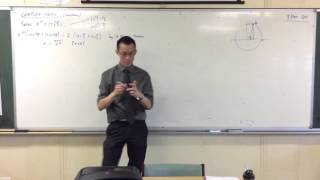Complex Conjugate Root Theorem (1 of 4: Using DMT and Polar Form to solve for Complex Roots)
3y5m ago
08:34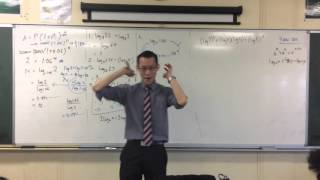Logarithms Review (4 of 4: Dealing with (logb)^2 and Logarithmic Quadratics)
3y5m ago
10:33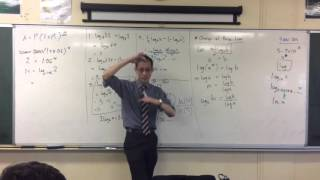Logarithms Review (3 of 4: Using Logs to answer Compound Interest Questions)
3y5m ago
09:39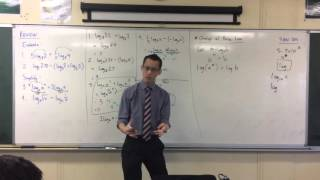Logarithms Review: (2 of 4: Proving the Change of Base Law)
3y5m ago
11:14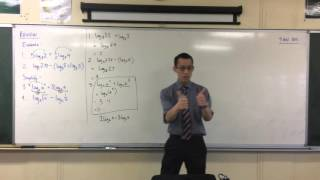Logarithms Review (1 of 4: Using Log Laws to solve Log Equations)
3y5m ago
05:18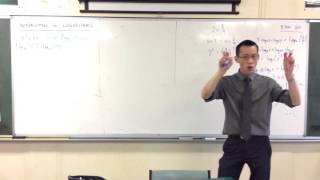Logarithmic Arithmetic (3 of 3: Using Logs to solve Exponential Equations)
3y5m ago
03:43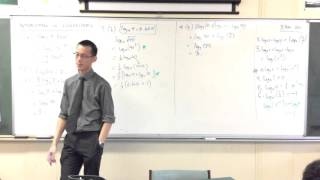Logarithmic Arithmetic (2 of 3: Harder Numerical Examples)
3y5m ago
08:12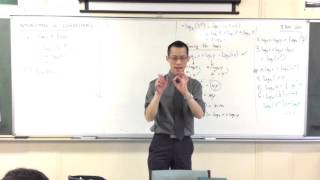Logarithmic Arithmetic (1 of 3: Basic Numerical Examples)
3y5m ago
06:21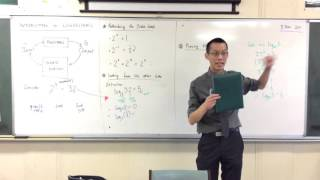Introduction to Logarithms (2 of 2: Definition & Basic Principles)
3y5m ago
07:58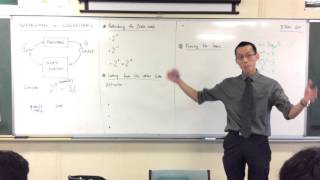Introduction to Logarithms (1 of 2: Rethinking Exponentials)
3y5m ago
10:28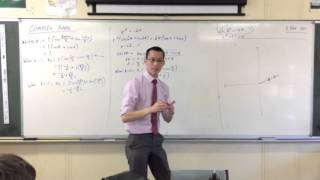Complex Roots (5 of 5: Flowing Example - Solving z^6=64)
3y5m ago
03:52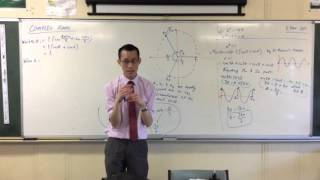Complex Roots (4 of 5: Through Polar Form Generating Solutions)
3y5m ago
09:51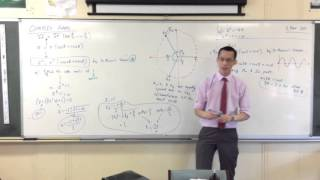Complex Roots (3 of 5: Through Polar Form Using De Moivre's Theorem)
3y5m ago
05:23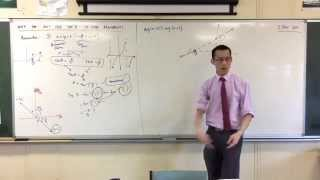Using Inverse tan to find arguments? (2 of 2: Why it works... Sometimes)
3y5m ago
11:51Using Inverse tan to find arguments? (1 of 2: Why it doesn't work... Sometimes)
3y5m ago
07:04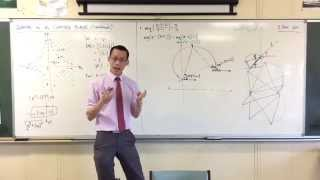Graphs on the Complex Plane (4 of 4: Exploring how the argument traced the graph)
3y5m ago
10:30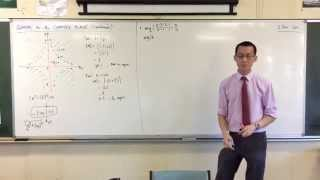Graphs on the Complex Plane (3 of 4: Geometry of arg(z)-arg(z-1))
3y5m ago
05:38Graphs on the Complex Plane [Continued] (2 of 4: Finding Regions of Inequality by Testing Points)
3y5m ago
11:56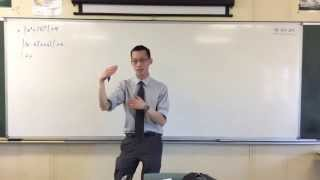Graphs on the Complex Plane [Continued] (1 of 4: What's behind the graph?)
3y5m ago
10:26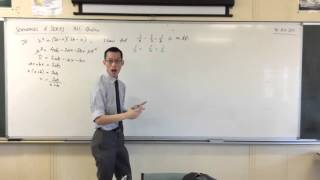Series and Sequences: HSC Question Help (2 of 2)
3y5m ago
11:00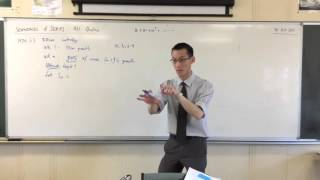Series and Sequences: HSC Question Help (1 of 2)
3y5m ago
13:18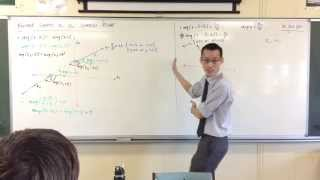Further Graphs on the Complex Plane (3 of 3: Geometrical Representation of Arguments)
3y5m ago